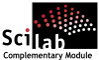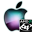ATOMS : Linear Algebra details

# Linear Algebra

A collection of algorithms for linear algebraDetails
Version
0.1
A more recent valid version with binaries for Scilab 5.3 exists: 0.2
Author
Michael Baudin
Owner Organization
DIGITEO
Maintainer
Michael Baudin
Category
Dependency
Creation Date
June 6, 2011
Source created on
Scilab 5.3.x
Binaries available on
Scilab 5.3.x:Linux 32-bitWindows 32-bitWindows 64-bitmacOSLinux 64-bit
Install command
`--> atomsInstall("linalg")`
Report a bugDescription
```            The goal of this toolbox is to provide a collection of
algorithms for linear algebra.

These algorithms are most of the time already provided by Scilab, but
are available here for comparison purpose.

The linalg_pow function is much faster than Scilab's pow,
when the exponent is large.
The algorithm used here is based on binary exponentiation, and
requires only log2(p) iterations, compared to p iterations for the
naive pow.
For example, for p=99999, it was found that linalg_pow requires 0.003
seconds for a 5-by-5 matrix, while Scilab requires 2.7 seconds.

The linalg_zhbev function provides the same feature as Lapack's
ZHBEV, that is, computes the eigenvalues of a complex hermitian band matrix.
The other method in Scilab to compute eigenvalues of sparse matrices
is to use Arnoldi's iterations.
This does not make use of the Hermitian structure of some matrices.

Features :
-------

* linalg_chol — Computes the Cholesky decomposition.
* linalg_condeig — Computes the condition number of the eigenvalues of a
matrix.
* linalg_expm — Computes the exponential of a matrix.
* linalg_factorlu — Computes the LU decomposition without pivoting.
* linalg_factorlupivot — Computes the LU decomposition with pivoting.
* linalg_gaussnaive — Solves a linear equation by Gauss method and no
pivoting.
* linalg_gausspivotal — Computes the solution of a linear equation with Gauss
and row pivoting.
* linalg_gausspivotalnaive — Computes the solution of a linear equation with
Gauss and row pivoting.
* linalg_hbandL — Converts a complex hermitian band matrix into its compact
form.
* linalg_pow — Computes A^p
* linalg_powfast — Computes A^p
* linalg_rayleighiteration — Computes approximated a pair of eigenvalue and
eigenvector.
* linalg_solvelu — Computes the solution of a linear equation, given its LU
decomposition.

BLAS/LAPACK
* linalg_dgemm — Computes D = Alpha*A*B+ Beta*C for a real matrix.
* linalg_dsyev — Computes the eigenvalues of a symmetric real matrix.
* linalg_zgemm — Computes D = Alpha*A*B+ Beta*C for a complex matrix.
* linalg_zhbev — Computes the eigenvalues of a complex hermitian band
matrix.
```Files (6)Source code archive
```
```Linux 32-bit binary for Scilab 5.3.x
```Linux 32-bit
Automatically generated by the ATOMS compilation chain

```Windows 32-bit binary for Scilab 5.3.x
```Windows 32-bit
Automatically generated by the ATOMS compilation chain

```Windows 64-bit binary for Scilab 5.3.x
```Windows 64-bit
Automatically generated by the ATOMS compilation chain

```macOS binary for Scilab 5.3.x
```MacOSX version
Automatically generated by the ATOMS compilation chain

```Linux 64-bit binary for Scilab 5.3.x
```Linux 64-bit
Automatically generated by the ATOMS compilation chain

```News (0)Comments (2)
[get_person] Le compte avec l'identifiant 2125 n'existe pas